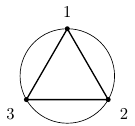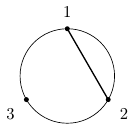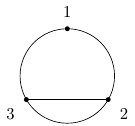Queries for Set partitions: search statistic / browse statistics / browse maps from / browse maps to

# Definition & Example

• A set partition of size $n$ is a partition of the set $\mathcal{S} = \{1,\ldots,n\}$. This is a collection of non-empty pairwise disjoint subsets (parts) of $\mathcal{S}$ whose union is $\mathcal{S}$. In symbols, $\mathcal{P} = \{P_1,\ldots,P_k \}$ such that

$$S = P_1 \sqcup P_2 \sqcup \dots \sqcup P_k, \quad P_i \cap P_j = \emptyset \text{ for all }i \neq j, \quad \emptyset \notin \mathcal{P}.$$

 the 5 Set partitions of size 3{{1,2,3}} {{1,2},{3}} {{1,3},{2}} {{1},{2,3}} {{1},{2},{3}}

• Set partitions of size $n$ are graphically represented by drawing the numbers $1$ through $n$ around a circle and then drawing the convex hulls of the blocks.

• The number of set partitions of size $n$ is $n$-th Bell number $B_n$ (A000110).

• The number of set partitions of size $n$ into $k$ blocks is the Stirling number of the second kind (A008277).

• The set partitions of size $n$ form a poset by containment order. This poset is indeed a lattice which is the intersection lattice of the braid arrangement.

• A set partition is said to be non-crossing if the graphical representation does not have any crossing blocks. In symbols, this is to say that there does not exist $P_i, P_j \in \mathcal{P}$ which contains elements $a, b \in P_i$ and $x, y \in P_j$ such that $a < x < b < y$. The number of non-crossing set partitions of size $n$ is the n-th Catalan number.

# Technical information for database usage

• A set partition is represented as a set of disjoint blocks, which are themselves sets.
• Set partitions are graded by the size of the ground set.
• The database contains all set partitions of size at most 7.

If you want to edit this wiki page, you can download the raw markdown and send your new version to info@findstat.org